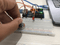Overview:

Light Emitting Diodes (LEDs) are popularly used in electronics application. These come in multiple colors such as blue, red, and green. It has been used as an indicator, illuminator, and has been incorporated with various sensors.  As such, the Upgraded Arduino Kit contains a number of LEDs. In this five part tutorial, the LED will be introduced and incorporated with the other components found in the Arduino kit.

In the first tutorial, the potentiometer is used to control the LED brightness.

Hardware:

You can buy all this Hardware at Createlabz.

Software:

Application Discussion

This configuration gives a similar overview as to how dimmers in modern homes operate. The dimmers in modern homes are connected to light fixtures which adjusts the brightness of the light. This is made possible by controlling the voltage applied to the light, thus, varying the intensity.

However, in this setup we make use of the Arduino Uno and adjust the brightness with the PWM output. The pins on the Arduino which support PWM output are the pins with the "~" mark next to them. The concept of using the PWM output instead of the digital is explained further in the code breakdown.

Arduino Uno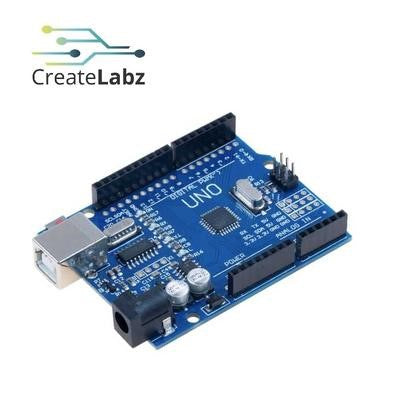The Arduino Uno is one type of the many Arduino boards developed by the Atmel Corporation. The Arduinos are mostly designed with the 8-bit AVR microcontroller. The Arduino Uno consists of 14 digital input/output pins, 6 analog input pins, a USB connection, a power barrel jack, an ICSP header, and a reset button.

The Arduino Uno is based on the ATmega328P microcontroller. Thus, serves as microcontroller board with the same functions. In order to program the board, Arduino IDE is required which utilizes a set of C/C++ functions.

Potentiometer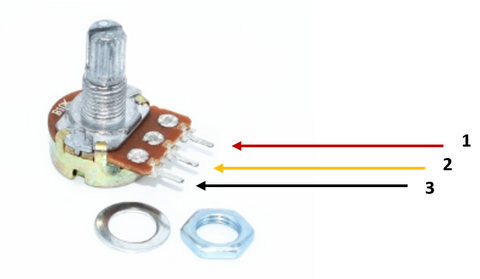The potentiometer has 3 pins for the ground, output, and voltage source. This is a component which varies the resistance through a knob. The resistance can be read using the Arduino board as an analog input.

 Pin No. Pin Name 1 VCC 2 Output 3 GND

There is a wide array of different potentiometer values, however some standard values are as follows: 500, 1K, 2K, 5K, and 10K. Potentiometers are usually used in audio devices to control its volume. It is also covered with a cap which makes it less distinguishable.

How it works: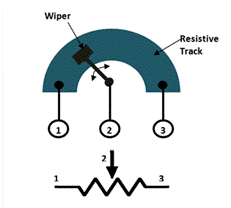The diagram above represents the inside of a potentiometer and its symbol. The middle pin, serves as the wiper which slides through the resistive track to vary the resistance. The value of the potentiometer is the complete resistance of the resistive track.

In order to measure its full value, simply take the resistance between the 1st and 3rd terminal. If the wiper were to be positioned exactly in the middle, measuring the resistance between terminal 1 and 2 will give 50% of the full value of the potentiometer. This case is the same in measuring from terminal 2 and 3.

Pulse Width Modulation

The Pulse Width Modulation technique is used to obtain an analog output using digital signals. The Arduino digital pins give off two states, the HIGH (5v) and the LOW (0V). These two states give off a square wave signal.If these two states were to be interchanged at a very fast rate, the output will seem continuous. An example would be the dimming of an LED. Such output reflects an analog signal.

To further understand, it is necessary to identify the terms used in PWM.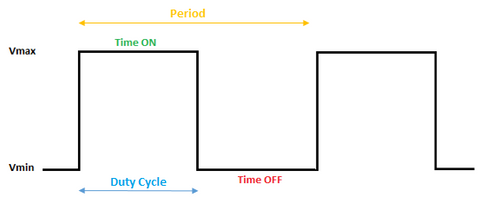Period – is one full repetition of the wave

Duty cycle – percentage of time when the signal is high in one period

In the figure shown above, assuming the duty cycle is 50% we get:

Vout = Vmax * Duty Cycle

Vout = 2.5 V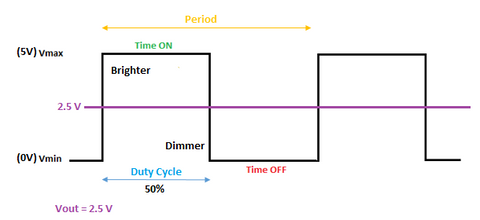As the duty cycle increases the voltage output also increases. Thus, results to a brighter output from the LED. The figure below shows the pulse width modulation from different duty cycles.In this tutorial, we will make use of the Arduino function analogWrite(pin, value) where pin is the Arduino pin where LED is connected, and value is a number from 0 to 255, which varies the duty cycle accordingly with value = 0 having 0% duty cycle and value = 255 having 100% duty cycle.

Set-up the hardware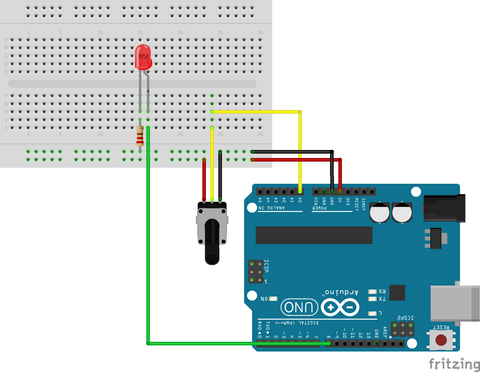Connecting the potentiometer

Connect the VCC and GND pins of the potentiometer to the 5V and GND pin of the Arduino, respectively. The output pin of the potentiometer, which is the middle pin, is then plugged in to the A0 pin.

Connecting the LED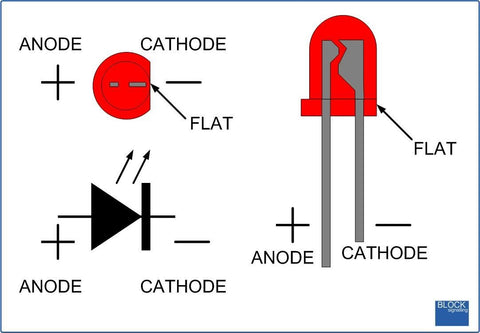The LED pins have noticeably different lengths. The longer leg is for the positive end and the shorter is for the ground. First, connect a 220 ohm resistor to the ground then to the shorter leg of the LED. The resistor serves as a current-limiting resistor to protect the LED from the full voltage. The longer leg of the LED is then connected to the pin 8 of the Arduino, which serves as the output.

Set-up the software

Open the Arduino IDE and upload the code on the Arduino.

The Arduino code can be found in Github here or you can copy the code below.

Arduino Code

``````const int POT = A0; //analog input pin for potentiometer
const int LED = 9; //input pin for LED

int POTvalue=0; //variable for input values from A0
int outPOTvalue=0; //variable for output value from potentio

void setup() {
//Initialize pinModes
pinMode (LED,OUTPUT);
pinMode (POT,INPUT);

}

void loop() {
outPOTvalue = map(POTvalue,0,1023,0,255); //re-map from 0-1023 to 0-250

analogWrite(LED,outPOTvalue);
delay(1000);
}
``````

Code Breakdown

• const int var
– specifies that the value assigned to the variable cannot be changed, thus, making it a read only variable

• int var
– creates a variable integer

• pinMode(pin,mode)
– Configures the identified pin to behave as an input or an output

• analogWrite
– turns the LED depending on the value of the outPOTvalue variable. It adjusts the brightness of the LED with 0 being the lowest and 255 being the highest.

The digital pins of the Arduino read only two states, the HIGH and LOW states. Thus, making it difficult to adjust the LED brightness. The HIGH (5V) and LOW (0V) states only make it possible to switch the LED on and off, respectively.

However, with the PWM output, the HIGH and LOW states can be varied using the analogWrite. From the code above it is necessary to map the read values from the potentiometer. The analogRead of the Arduino ranges from 0-1023 but the analogWrite can only range from 0-255. Therefore, the analogRead values are then mapped down to a lower range. This then allows the output of the LED to be varied from 0-255. This is very different from having only the HIGH and LOW states from the digital output.

Conclusion

No matter how simple an Arduino project is, it may be a representation of an everyday feature in your daily life. With the use of 3 components, the replication of a simple dimmer was made possible. This simple project on the Arduino Uno can already serve as a basic introduction on how it operates.

There are many possible projects you can do with the Arduino Uno that also makes use of minimal components. If you want to try making similar project such as this with a different input, you can try to use a photoresistor to dim an LED.  Another project you may also want to try is using the potentiometer to control the LED as a level indicator.

Check out other projects in this Controlling an LED series:

Adjusting LED brightness using a Photoresistor

Turning on/off multiple LEDs and buzzer

Music reactive LEDs using Sound Module

References

https://components101.com/microcontrollers/arduino-uno